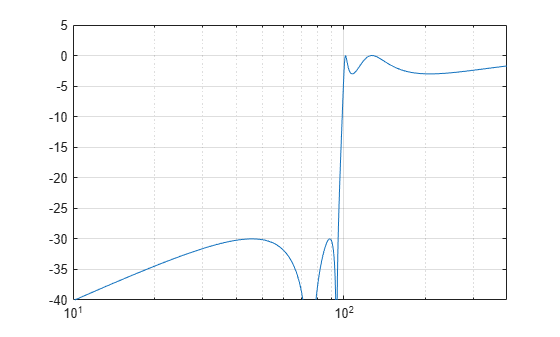Documentation

lp2hp

Transform lowpass analog filters to highpass

Description

example

[bt,at] = lp2hp(b,a,Wo) transforms an analog lowpass filter prototype given by polynomial coefficients (specified by row vectors b and a) into a highpass analog filter with cutoff angular frequency Wo. The input system must be an analog filter prototype.

[At,Bt,Ct,Dt] = lp2hp(A,B,C,D,Wo) converts the continuous-time state-space lowpass filter prototype (specified by matrices A, B, C, and D) to a highpass analog filter with cutoff angular frequency Wo. The input system must be an analog filter prototype.

Examples

collapse all

Design a 5th-order highpass elliptic filter with a cutoff frequency of 100 Hz, 3 dB of passband ripple, and 30 dB of stopband attenuation

Design the prototype. Convert the zero-pole-gain output to a transfer function.

f = 100;

[ze,pe,ke] = ellipap(5,3,30);
[be,ae] = zp2tf(ze,pe,ke);

Transform the prototype to a highpass filter. Specify the cutoff frequency in rad/s.

[bh,ah] = lp2hp(be,ae,2*pi*f);

Compute and plot the frequency response of the filter. Divide the normalized frequency by $2\pi$ so the x-axis of the plot is in Hz.

[hh,wh] = freqs(bh,ah,4096);

semilogx(wh/2/pi,mag2db(abs(hh)))
axis([10 400 -40 5])
gridInput Arguments

collapse all

Prototype numerator and denominator coefficients, specified as row vectors. b and a specify the coefficients of the numerator and denominator of the prototype in descending powers of s:

$\frac{B\left(s\right)}{A\left(s\right)}=\frac{b\left(1\right){s}^{n}+\cdots +b\left(n\right)s+b\left(n+1\right)}{a\left(1\right){s}^{m}+\cdots +a\left(m\right)s+a\left(m+1\right)}$

Data Types: single | double

Prototype state-space representation, specified as matrices. The state-space matrices relate the state vector x, the input u, and the output y through

$\begin{array}{l}\stackrel{˙}{x}=Ax+Bu\\ y=Cx+Du\end{array}$

Data Types: single | double

Cutoff angular frequency, specified as a scalar. Express the cutoff angular frequency in rad/s.

Data Types: single | double

Output Arguments

collapse all

Transformed numerator and denominator coefficients, returned as row vectors.

Transformed state-space representation, returned as matrices.

Algorithms

lp2hp transforms analog lowpass filter prototypes with a cutoff angular frequency of 1 rad/s into highpass filters with a desired cutoff angular frequency. The transformation is one step in the digital filter design process for the butter, cheby1, cheby2, and ellip functions.

lp2hp is a highly accurate state-space formulation of the classic analog filter frequency transformation. If a highpass filter is to have a cutoff angular frequency ω0, the standard s-domain transformation is

$s=\frac{{\omega }_{0}}{p}.$

The state-space version of this transformation is:

At = Wo*inv(A);
Bt = -Wo*(A\B);
Ct = C/A;
Dt = D - C/A*B;

See lp2bp for a derivation of the bandpass version of this transformation.